# How do I do the prime factorization

## Prime factorization / prime factors

We explain the prime factorization here. Let's look at this:

• A Explanationwhat prime factors are and how to do prime factorization.
• Lots Examples with numbers.
• A Video to this topic.
• A Question and answer area to this area.

Tip to start: In order to be able to carry out the prime factorization yourself, you have to know what a prime number is and you should know the rules of divisibility. I will briefly explain both here. If this is not enough, please have a look at the main article on prime numbers and divisibility rules.

### Explanation of prime factorization

Prime factorization is about breaking up a number into small prime numbers and multiplying them together. What was prime again? Well, a prime number is a natural number that is only divisible by itself and by 1 with no remainder. However, the 1 has been excluded. The first prime numbers are 2, 3, 5, 7, 11, 13, 17, 19, 23, 29, 31, 37, 41, 43, 47, 53.

Divisibility:

In order to be able to decompose a number, you still need knowledge of divisibility. Is a number divisible by another number without a remainder? 6: 2 = 3. So no remainder. 7: 2 = 3 Remainder 1. So we have a remainder.

Note:

The first and most important divisibility rules are:

• A number is divisible by 2 if the ones digit is 2, 4, 6, 8 or 0.
• A number is divisible by 3 if the checksum is divisible by 3.
• A number is divisible by 5 if the ones digit is 0 or 5.

More rules for larger numbers and examples under rules of divisibility.

Why do you need prime factorization?

The decomposition into prime factors is needed, for example ...

• ... for the determination of the LCM (small common multiple)
• ... for the determination of the GCD (greatest common factor)
• ... in fractions (main denominator and abbreviation)
• ... for encryption methods in data traffic

What are prime factors?

These are prime numbers that are multiplied together. Hopefully everyone still knows the word factor from multiplications: first factor times second factor equals product, for example 3 · 4 = 12.

Display:

### Examples of prime factorization

Let's prime some numbers. We use it to generate smaller numbers that are multiplied together. To do this, we take small prime numbers and try to divide the initial number by them without leaving a remainder.

example 1:

The number 36 is to be broken down into prime factors. What does the calculation and the result look like?

Solution:

As a reminder, the first prime numbers again. These were 2, 3, 5, 7, 11 etc. We now take the starting number 36 and try to divide it by 2 first. This also works without a remainder, because 36: 2 = 18. So the prime factorization looks like this up to now: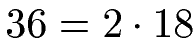Now we are checking whether further decomposition is possible. To do this, we look at 18 and check whether it is divisible by 2. This is also possible because 18: 2 = 9.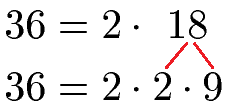Can we still disassemble the 9? Let's try 2 again. Then we get 9: 2 = 4 remainder 1. We have a remainder. So let's try the next prime, which is 3. With 9: 3 = 3 this also works without a remainder. We only have prime factors on the right-hand side of the equation. We're done with that. The complete decomposition into prime factors looks like this: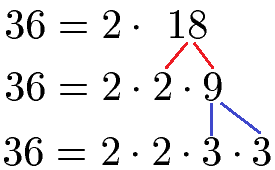Example 2:

The number 450 is to be broken down into prime factors.

Solution:

We take 450 and try to divide by 2 first. This is also possible, then the 450 ends in the number 0 and is therefore divisible by 2 without a remainder. With 450: 2 = 225 we take the first step.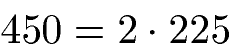Can we disassemble the 225? Certainly not with a 2, then 225 ends in a 5 and is therefore not divisible by 2 without a remainder. So we try the next prime number, the 3. This works because the checksum of 225 is 2 + 2 + 5 = 9. And 9 is divisible by 3 without a remainder. We can therefore break the 225 down into 3 * 75.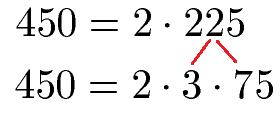We cannot divide 75 by 2 without a remainder. By 3, however, we can, since 75 = 3 · 25.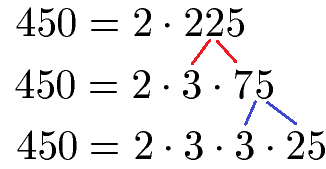We can neither divide 25 by 2 nor by 3 without a remainder. However, it goes through the next prime number - the 5. We thus get 25 = 5 · 5. This completes the decomposition into prime factors. The finished calculation looks like this: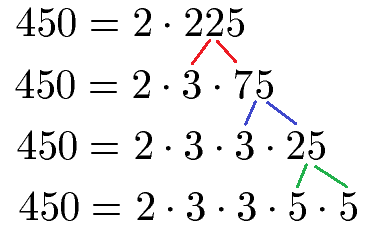Example 3 with prime factor tree:

If the numbers get bigger (well over 100 or even over 1000) you can use a tree to break down the number into its prime factors. This should be shown once with the number 700. First the complete tree with calculation method, then this is explained.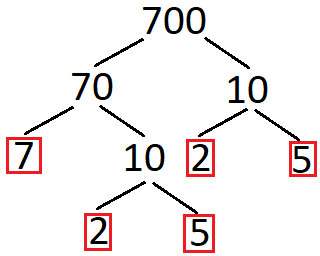In principle, we take the starting number and always try to build smaller multiplications. For the start 700 = 70 · 10. We break this apart until we only have prime numbers. If we reach a prime number, we circle it in red. This then results in: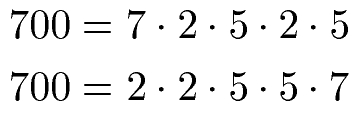Show:

### Examples

In the next video you will be introduced to the following content on prime factorization and related topics:

• What is a prime number?
• How do you perform a prime factorization?
• Examples of prime factorization.

Next video »